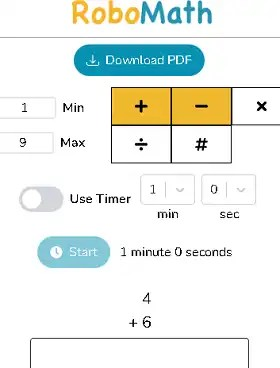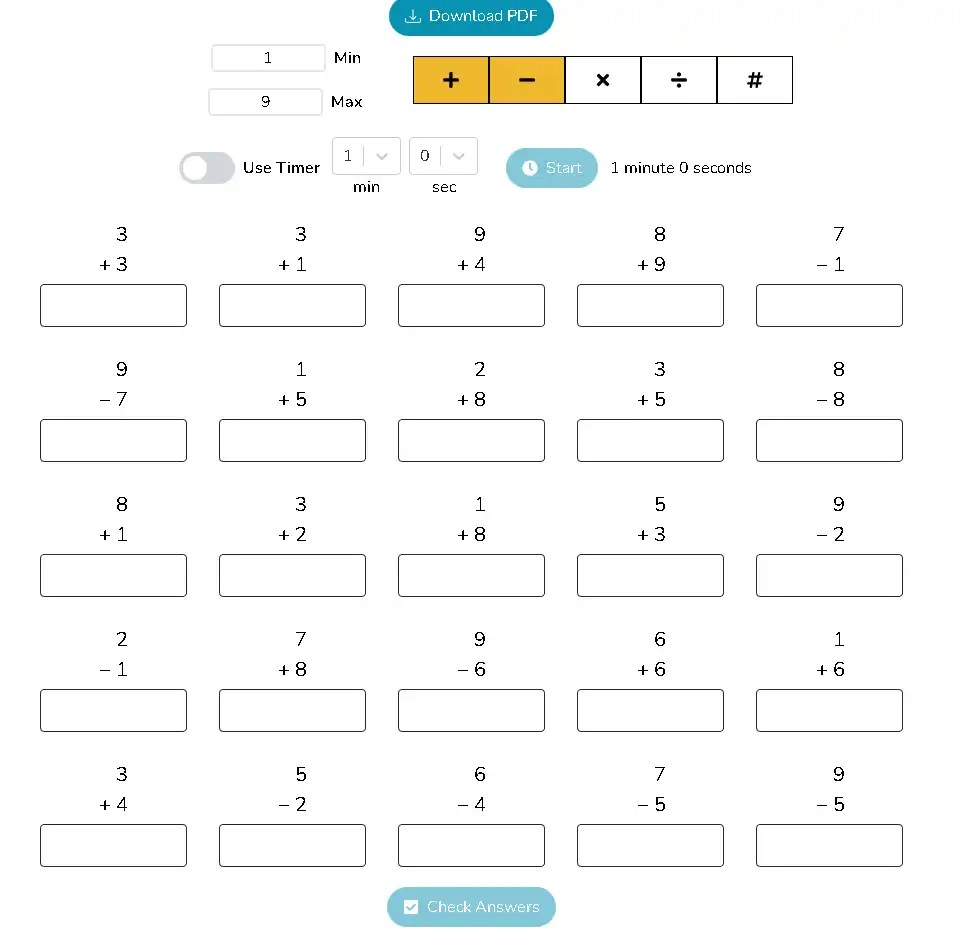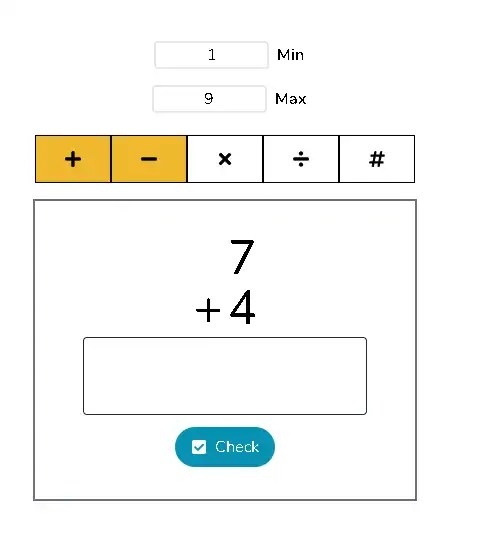# RoboMath : Online Tool to learn and Practice Math

Posted by

RoboMath is a website where you can use worksheets and flashcards to learn and practice math online. Kevin Mach, a software engineer, made this easy-to-use, fun, and free tool so that everyone can learn math.

If you or your children need help with math or you just can’t seem to find a way to make math fun, RoboMath is the solution. If you want to get better at mental arithmetic and want to put your multiplication skills to the test, then this website is the place to do it.

This online tool was made to give you a place where you can focus on learning math. So, the platform gives you the chance to test your mathematical skills with a variety of activities. The first one is in a spreadsheet format. It is a series of 25 simple and randomly generated math operations, and you can try addition, subtraction, division, or multiplication.

You can choose one operation at a time or several at once. RoboMath then does calculations based on the operations you chose and the number range you set (which can be between 1 and 9999).You can also set a timer and try to do all the calculations in the time given to make the task more challenging. Once you’ve done all the calculations, click the “Check” button. The tool checks your answers, so if you made a mistake, you can go back and fix it.

In addition to worksheets, flashcards can be used for practice. This feature displays the problems one by one. A fun way to test your abilities is to see how many calculations you can accomplish in a given time limit.Lastly, this website lets you download the worksheets in PDF format for free. That way, you can easily print out the worksheets and give them to your kids or students. Simply put, this is an excellent resource for developing mathematical skills.

Go ahead and test your Math skills at   https://robomath.tech/